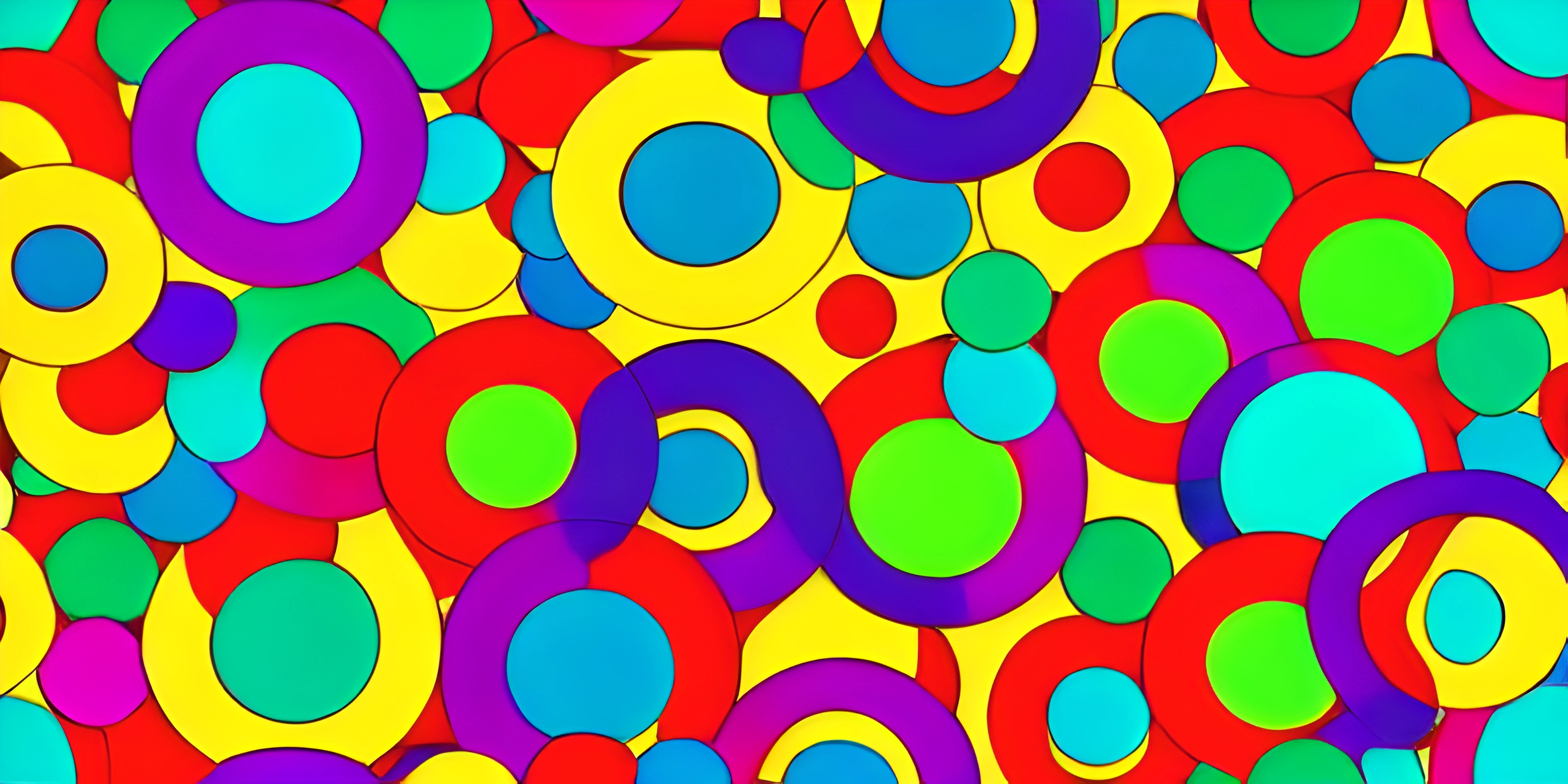# p5.js ColorsNote: this page has been created with the use of AI. Please take caution, and note that the content of this page does not necessarily reflect the opinion of Cratecode.

Colors are the rainbows of the programming world, and p5.js makes it incredibly easy to add vibrant hues to your digital canvas. This guide will take you on a journey through the world of colors in p5.js, showing you how to create, manipulate, and manage colors in your sketches.

## Getting Started: Basic Colors

In p5.js, a color is represented as an object. The most common method for creating a color object is by using the `color()` function, which accepts three or four arguments, corresponding to the RGBA color model.

Here's an example:

``let myColor = color(255, 0, 0);``

This creates a bright red color object, with the RGB values (255, 0, 0). If you'd like to include the alpha (transparency) value, simply add a fourth argument:

``let myColor = color(255, 0, 0, 128);``

Now, `myColor` represents a semi-transparent red.

## Named Colors

p5.js also provides a handful of predefined, named colors. These can be accessed using the `color()` function with a single string argument representing the color name. For example:

``let skyBlue = color("skyblue");``

This creates a color object representing the sky blue color.

## Color Modes

By default, p5.js uses the RGB color mode, but you can switch to the HSL color mode using the `colorMode()` function:

``````colorMode(HSL);
let myColor = color(120, 100, 50);``````

This creates a color object with the HSL values (120, 100, 50), which represents a bright green color.

## Setting Colors

Once you have your color objects, you can use them to set the fill and stroke colors in your sketch. The `fill()` and `stroke()` functions accept color objects as arguments:

``````let red = color(255, 0, 0);
let green = color(0, 255, 0);

fill(red);
stroke(green);

rect(50, 50, 100, 100);``````

This draws a red square with a green border.

## Manipulating Colors

p5.js provides several functions to manipulate colors, such as `lerpColor()` for interpolating between two colors or `brightness()` to get the brightness value of a color. Here's an example that uses `lerpColor()`:

``````let startColor = color(255, 0, 0);
let endColor = color(0, 255, 0);

let interpolatedColor = lerpColor(startColor, endColor, 0.5);

fill(interpolatedColor);
rect(50, 50, 100, 100);``````

This draws a square with a color halfway between red and green.

## Conclusion

Color is a fundamental aspect of any visual project, and p5.js makes it easy to create and manage colors in your sketches. By understanding the basics of creating and manipulating color objects, you'll be able to bring your digital canvas to life with vibrant hues and captivating visuals. Happy coloring!

## FAQ

### What are the basics of using colors in p5.js?

In p5.js, you can create colors using the `color()` function, which accepts either RGB, RGBA, HSB, or HSL values. To set the fill or stroke color, you can use the `fill()` and `stroke()` functions respectively. Here's a basic example:

``````function setup() {
createCanvas(400, 400);
}
function draw() {
background(220);
// Set fill color
fill(255, 0, 0);

// Set stroke color
stroke(0, 0, 255);
// Draw a circle with a red fill and blue stroke
circle(width / 2, height / 2, 100);
}``````

### How do I switch between RGB and HSB color modes?

You can switch between color modes using the `colorMode()` function. The default mode is RGB, but you can set it to HSB by passing `HSB` as an argument. For example:

``````function setup() {
createCanvas(400, 400);
colorMode(HSB);
}
function draw() {
background(0);

// Set fill color in HSB mode
fill(100, 100, 100);

// Set stroke color in HSB mode
stroke(200, 100, 100);
// Draw a circle with the new colors
circle(width / 2, height / 2, 100);
}``````

### Can I create gradients using p5.js colors?

Yes! You can create gradients by interpolating between two or more colors using the `lerpColor()` function. Here's an example of a simple linear gradient:

``````function setup() {
createCanvas(400, 400);
}
function draw() {
background(220);
let color1 = color(255, 0, 0);
let color2 = color(0, 0, 255);

for (let i = 0; i < width; i++) {
let gradientColor = lerpColor(color1, color2, i / width);
line(i, 0, i, height);
}
}``````

### How can I create translucent colors in p5.js?

To create a translucent color, you can use the `color()` function with an additional alpha parameter (0-255 range), or you can use the `alpha()` function to set the alpha value for an existing color. For example:

``````function setup() {
createCanvas(400, 400);
}
function draw() {
background(220);

// Create a translucent red color
let translucentRed = color(255, 0, 0, 128);
fill(translucentRed);

// Draw a translucent red circle
circle(width / 2, height / 2, 100);
}``````